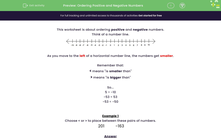# Order Positive and Negative Numbers

In this worksheet, students will use the < and > symbols to order positive and negative numbers.Key stage:  KS 2

Curriculum topic:   Number: Number and Place Value

Curriculum subtopic:   Count Backwards (including Negative Numbers)

Difficulty level:#### Worksheet Overview

This activity is about ordering positive and negative numbers.

Think of a number line.As you move to the left of a horizontal number line, the numbers get smaller.

Remember that:

<  means "is smaller than"

>  means "is bigger than"

So...

5 > -10

-53 < 53

-53 < -50

The further to the left of zero we get on a number line, the smaller the number.

Think about the reading on a thermometer on a really cold winter's day! If the temperature outside is -3, it's pretty cold, but if it goes down to -10, then it is even colder! -10 is colder than -3 because it is further away from zero.

Example 1

Choose < or > to place between these pairs of numbers.

201 -163

201 is positive.

-163 is negative.

Positive numbers are always bigger than negative numbers.

So 201 is bigger than -163.

201
 >
-163

Example 2

Choose < or > to place between these pairs of numbers.

-201 -163

-201 is negative.

-163 is negative.

Which is further to the left on the number line?

-201 is more negative, so it is smaller than -163.

So -201 is smaller than -163.

-201
 <
-163